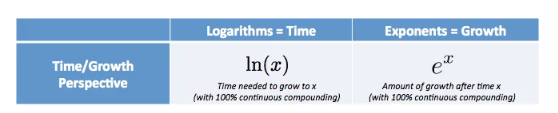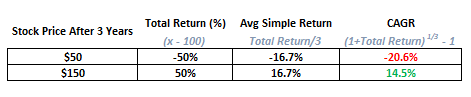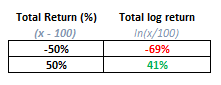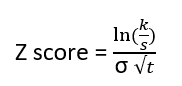# Using Log Returns And Volatility To Normalize Strike Distances

## Basic Review

Consider a \$100 stock. In a simple return world, \$150 and \$50 are each 50% away. They are equidistant. But in compounded return world they are not. \$150 is closer. This blog post will progress from an understanding of natural logs to normalizing the distance of asset strikes.

The use of log returns in financial and derivatives modeling is useful because investing contexts usually involve re-investing your capital. In other words, the growth process is multiplicative, not additive. But if it’s multiplicative we find ourselves needing to specify a compounding interval. This is an invitation to attach a cumbersome asterisk to every model.

Logarithms offer an elegant solution — they allow us to standardize an assumption:  returns are continuously compounded.

If you are uncomfortable already, these short primer posts will help you catch up. And don’t worry, we will revisit HS math intuitively in this post before getting to the main course.

• In Examples Of Comparing Interest Rates With Different Compounding Intervals, we saw how to convert back and forth between simple returns and compounded returns by dividing a holding period into different intervals.
• In Understanding Log Returnswe showed how log returns are an extreme case of compounded returns — it assumes that compounding occurs continuously. In other words as you divide the holding period into smaller and smaller intervals, you find a rate that is smaller than the growth rate for the entire holding period. If the growth from \$1 to \$2 is fixed than the more compounding periods there are, the lower the rate must be in order for \$1 to end up being \$2.

You probably remember hearing about the constant e and the natural log from math class. You also repressed it. Because it was taught poorly.

Understanding e

We’ll turn to betterexplained.com:

e is NOT just a number!

Describing e as “a constant approximately 2.71828…” is like calling pi “an irrational number, approximately equal to 3.1415…”. Sure, it’s true, but you completely missed the point. Pi is the ratio between circumference and diameter shared by all circles. It is a fundamental ratio inherent in all circles and therefore impacts any calculation of circumference, area, volume, and surface area for circles, spheres, cylinders, and so on.

e is the base rate of growth shared by all continually growing processes. e lets you take a simple growth rate (where all change happens at the end of the year) and find the impact of compound, continuous growth, where every nanosecond (or faster) you are growing just a little bit.

e shows up whenever systems grow exponentially and continuously: population, radioactive decay, interest calculations, and more.

Just like every number can be considered a scaled version of 1 (the base unit), every circle can be considered a scaled version of the unit circle (radius 1), and every rate of growth can be considered a scaled version of e (unit growth, perfectly compounded).

So e is not an obscure, seemingly random number. e represents the idea that all continually growing systems are scaled versions of a common rate.

Let’s say our basic unit of time is a year.

e is the constant that says “if I start with \$1 and continuously compound at a rate of 100%, how much do I end up with…\$2.71828”

Understanding the natural logarithm (ln)

It’s true that the natural log is the inverse of an exponential of base e just as logs answer the question “what power do I raise 10 to in order to get to X?”. But defining the natural log as an inverse is circular not intuitive. Again, we turn to BetterExplained. From Demystifying the Natural Logarithm (ln):

The natural log gives you the time needed to reach a certain level of growth.e and the Natural Log are twins:

ex is the amount we have after starting at 1.0 and growing continuously for x units of time

ln⁡(x) is the time to reach amount x, assuming we grew continuously from 1.0

If e is about growth, the natural log (ln) is about how much time it takes to achieve that growth.

The Natural Log is About Time

• ex lets us plug in time and get growth.
• ln(x) lets us plug in growth and get the time it would take.

For example:

• e3 is 20.08. After 3 units of time, we end up with 20.08 times what we started with.
• ln⁡(20.08) is about 3. If we want growth of 20.08, we’d wait 3 units of time (again, assuming a 100% continuous growth rate).

Let’s apply e and natural logs to asset returns to understand how to normalize distances.

## Normalizing Distance

Let’s return to the \$100 stock. We said \$150 is closer than \$50 in the world of compounding. Let’s assume our growth occurs over 3 years. Here’s a summary of simple returns vs annually compounded returns (or CAGR):So far so good. The compounded returns are lower than the simple average return. Since log returns are just compounded returns sampled continuously we’d expect them to be even lower.

The total log return is indeed lower than the total simple return.We can also see that in logspace -50% total return is “further” away than up 50%. This is the first encounter we get with the concept of distance where we see that 50% in either direction is not the same. But by the end of this post, you will learn how to normalize even 2 log returns that look the same, but don’t mean the same thing.

But before that, we will need to complete our understanding of log returns. We saw that the 3-year total log returns are lower than the 3-year total returns. To do that I pose the question:

Can you compute the annualized log returns?

Pattern-matching the computations for average simple returns and CAGR, it appears we have 2 choices respectively:

1. Total log return / 3or
2. (1 + Total log return) 1/3 – 1

Remember what e and ln mean in the first place:

The expression ex is a total quantity of growth. It’s actually assumed to be e 1 * x where the 1 represents 100% continuously compounded growth and X represents a unit of time. The natural log or ln(ex) then solves for how much time (ie x) did it take to arrive at the total quantity of growth assuming 100% continuous compounding.

A key insight is that we don’t need to assume a 100% rate and x to be time. We can simply think of x as the product of “rate multiplied by time”. This allows us to substitute any rate for the assumed rate of 100% to find the time. Once again we turn to BetterExplained:We can use their logic to return to our question: Can you compute the annualized log returns from these total 3-year  log returns?Down Case:

log return = -69%

rate x time = -69%

rate x 3 = -69%

The annualized rate must be -23.1%

To annualize log returns, we simply take the total log return and divide by the number of years!

The complete summary table:All is right in the world…the more compounding intervals we divide the total period into the lower the return must be. Continuous compounding represents the most intervals we can slice the period into and therefore it is the smallest rate.

Recapping so far:

• Compounded rates are lower than simple rates for the same total return
• Log returns are convenient measuring sticks because we just assume continuous compounding
• etells us how much continuously compounded growth we get if we know the time period and rate
• The natural log can tell us:
• How much time we needed at a given rate to achieve that egrowth
• What rate we needed for a given time period to achieve that egrowth

## Normalizing Distances For Volatility

Let’s return to the \$100 stock and assume continuous compounding. What price on the downside is the equivalent of the stock moving up \$20? By now, we understand, the equivalent downside move is less \$20 away. Let’s compute the equivalent distances in log space.

ln(120/100) = 18.23%

We solve for a negative 18.23% log return:

ln(x/100) = -18.23%

x/100 = e-18.23%

x = .8333 * 100 = \$83.33

If the stock starts at \$100 then \$120 and \$83.33 are equidistant in log space.

We want to take this further. To compare distances, especially in different assets, we want to normalize for volatility.

Volatility is just another word for standard deviation. A 10% log return in BTC means a lot less than a 10% log return in 5-year Treasury notes. We should measure log returns in terms of how many standard deviations away a specified amount of growth is. Note, this is exactly what the concept of a z-score is in statistics. It tells us how far away from the mean a particular observation is.

Let’s stick with our \$100 stock and give it a volatility of 18.23%.

• A 1 standard deviation move to the upside in 1 year is \$120
• A 1 standard deviation move to the downside in 1 year is \$83.33

If we define K as a strike price, we can back into a general formula for how far K is from the spot price in terms of standard deviations. Let’s define all our variables first:

K = strike price

S = Spot price

σ = volatility

t = time (in years)

We start with an intuitive expression for a Z-score using our variables:We can confirm this makes sense with numbers from the previous example. We’ll set t to 1 (ie 1 year) and the Z-score is 1 corresponding to 1 standard deviation:The formula makes sense. In English, it says “divide the distance in logspace by the annualized volatility scaled to 1 year”.

This simply validated the expression for Z-score. We still want to define any strike price, K, as a function of its volatility and time.

Algebra ensues:• If you input a positive volatility number, the formula spits out what a 1 standard deviation up move is.
• If you input a negative volatility number, the formula spits out what a 1 standard deviation down move is.

If you recall, the big insight from earlier:

The expression ex is a total quantity of growth…we don’t need to assume a 100% rate and x to be time. We can simply think of x as the product of “rate multiplied by time”.

This fact can allow us to decompose the Z-score expression to account for the fact that our underlying stock process has both:

1. a drift component (option theory uses the risk-free rate for reasons that are beyond this post)
and
2. a random component drawn from a distribution defined by a mean (spot + drift) and volatility.

Defining the expressions:

• Risk-free rate or drift = r
• The mean of the distribution (aka the “forward”) = Sert
• The standard deviation scaled to time = σ√t

The Z-score formulas that incorporate drift for 1 standard deviation up and down respectively:

• Kup = Se(rt + σ√t)
• Kdown = Se(rt – σ√t)

[The rate in the ex portion is part drift and part random. Why do we combine them with addition instead of multiplication? Because the time portion affects each component differently. We can’t double the variance and halve the time because time also factors into the drift (ie the interest rate)]

Let’s wrap with an example, this time including the drift.

Set r = 5% and t = 1

Fwd = 100e.05 = \$105.13

If we are just considering the one standard deviation around the mean (as opposed to a full standard deviation up or down) this is the theoretical stock distribution:## What’s the point of all this?

For anyone within sneezing distance of a derivatives desk, these are rudiments. These computations are the meaning behind the Black Scholes’s z-scores (d1 and d2) and probabilities. These standardizations are critical for comparing vol surfaces. If you can’t contextualize how far a price is you cannot make meaningful comparisons between option volatilities and therefore prices.

If you only trade linear instruments because you are a well-adjusted human then hopefully you still found this lesson helpful. Seeing math from different angles is like filling in the grout in the tiles of your mental processing. You can measure the distance (or accumulated growth, positive or negative) in log space to account for compounding. You can standardize comparisons by using the asset’s vol as a measuring stick. And after all that, if you still don’t enjoy this, you can feel better about your life choices to do work that doesn’t rely on it.

If you do rely on understanding this stuff, hopefully you got e.00995-1 better today.